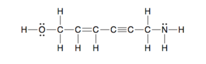# Problem: Count the total number of σ bonds and π bonds in the compound below:

🤓 Based on our data, we think this question is relevant for Professor Duis' class at NAU.

###### Problem Details

Count the total number of σ bonds and π bonds in the compound below:What scientific concept do you need to know in order to solve this problem?

Our tutors have indicated that to solve this problem you will need to apply the Sigma and Pi Bonds concept. You can view video lessons to learn Sigma and Pi Bonds. Or if you need more Sigma and Pi Bonds practice, you can also practice Sigma and Pi Bonds practice problems.

What is the difficulty of this problem?

Our tutors rated the difficulty ofCount the total number of σ bonds and π bonds in the compoun...as low difficulty.

How long does this problem take to solve?

Our expert Organic tutor, Jonathan took 1 minute and 55 seconds to solve this problem. You can follow their steps in the video explanation above.

What professor is this problem relevant for?

Based on our data, we think this problem is relevant for Professor Duis' class at NAU.

What textbook is this problem found in?

Our data indicates that this problem or a close variation was asked in Organic Chemistry - Klein 1st Edition. You can also practice Organic Chemistry - Klein 1st Edition practice problems.